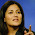## 5.01.2011

### Statistical Linear Tolerance Analysis

Tolerance
The total amount a specific dimension or parameter may vary

Tolerance Analysis
To predict the accumulation of tolerances in an assembly or other system

Tolerance analysis is a continued focus of the manufacturing industry as it seeks to both improve the quality of its products and produce them with lower cost. The appropriate allocation of tolerances in an assembly may result in lower costs per assembly and a higher probability of good fit, reducing the number of rejects or the amount of rework.
Statistical tolerance analysis is a powerful analytical method because it not only predicts the effect of manufacturing variation on design performance and production cost, but also allows designers and manufacturing personal to take advantage of statistical averaging to relax the component tolerances without sacrificing quality.

The variation on the workpieces, can come from differenct sources, such as:
• The machines which perform operations on the workpiece may have inherent inaccuracies built into them.
• The tools used on the machines are subject to dulling, general wear, chipping, breaking, and differences occurs due to regrinding
• The material used is subjected to variations
• The involvement of human elements
• Any other cause by Chance.

The tolerance analysis can be divided in two general types, Linear and Non-linear. This division depends on the transfer function that describes the tolerance value of the system under analysis. In the Linear Transfer functions the coefficients for the Xs are constant. (I.e. Vector loop, cycle time stack-ups, cost calculations)

Y = X1 + X2 - X3 - X4 + X5
Y = X1 + 2 X2 - 5 X3

Non-Linear tolerance transfer functions are defined when the coefficients for the Xs are functions of X. (i.e. geometric functions, performance predictions, response surface regressions)

In this article, we will review and compare two methods of linear tolerance analysis, Worst Case and Root Sum of Squares (RSS). The basic example we will use, applies to many domains, such as simple mechanical assemblies, cycle time calculations, cost predictions and in the DFSS methodology, and can be depicted as follows:

A vector loop is defined, according to common standards, the vector starts at the left surface      of the gap, in the same way, vectors from left to right are defined as positive (+), right to left as negative (-).
The transfer function for the Gap is defined as follows:

Gap Y = - Xblock4 - Xblock3 - Xblock2 - Xblock1 + Xenvelope

For this 4 blocks example:
• Blocks          1.2400 ± 0.003
• Envelope       4.9725 ± 0.003
• Thus, gap     0.0125 ± 0.0125

Worst Case Analysis Method.
This method is used to establish the extreme limits of Y. Worst-case analysis assumes ALL parts lie within spec and at their “worst” limits. The process to calculate it is:
1. Calculate Ynom by substituting nominal values of Xs into the transfer function
2. Calculate worst case tolerance tolY = tol1 + tol2 + ... + toln
3. Calculate worst case limits:
4.  Worst case maximum        = Ynom + tolY
5. Worst case minimum         = Ynom - tolY

Then:
Ynom= - Xblock4 - Xblock3 - Xblock2 - Xblock1 + Xenvelope
Ynom = - 1.24 - 1.24 - 1.24 - 1.24 + 4.9725 = 0.0125

tolY= tolblock4 + tolblock3 + tolblock2 + tolblock1 + tolenvelope
tolY = 0.003 + 0.003 + 0.003 + 0.003 + 0.003 = 0.015

Thus, worst case limits are.
Max    = Ynom + tolY = 0.0125 + 0.015 = 0.0275
Min     = Ynom - tolY = 0.0125 - 0.015= -0.0025

Worst case tolerance analysis, when used for tolerance allocation, often results in tight (and hence, expensive) tolerances on part dimensions.

Root sum of Squares analysis method.
Root sum squares (RSS) is a statistical method for computing the standard deviation of the response from the standard deviations of the X’s. The following conditions should be met:
1. The transfer function is linear.
2. The factors Xi are independent.

Normality of the X’s is not required.  If conditions 1 and 2 are met, RSS computes the correct standard deviation of Y even for non-normal X’s.  It’s often assumed that Y is normally distributed, for computing DPMO and Z values, but this assumption is unrelated to the RSS method.  For larger numbers of independent X’s in a linear function, Y’s distribution will approach normality by the Central Limit Theorem, even for non-normal X’s.

Then, for our example:
µY = - µblock4 - µblock3 - µblock2 - µblock1 + µenvelope
µY = - 1.24 - 1.24 - 1.24 - 1.24 + 4.9725
µY = 0.0125

Calculate the standard deviation of Y (assuming all std dev = 0.001):

Assume Y is normally (usually safe assumption if system has more than a few components, due to central limit theorem):

If we compare this tolerance analysis with the results from the worst case method we can prove the Worst Case can be extremely conservative.

This simple example is useful to compare both methods, and it is developed under some assumptions to make it simpler. It is important to remember that tolerances in the parts can be asymmetrical, the Xs in the transfer function can be correlated and the distribution of the tolerances can follow different distributions other than the uniform or normal distributions. Should you have any question regarding Tolerance Analysis, don’t hesitate to contact the consultants at TeraSigma Consulting, The Power of Collaboration.

#### 1 comment:

1.A statistical tolerance analysis is when you take the variation of a set of ... and all performance characteristics have a linear relationship with the dimension. feng shui